# Please clearly state your assumptions, number the equations and indicate logical connections between different lines.

## 1.                  [2+2 pt] Gaussian wavepackets and expectation values

Ohanian, #2.15, p. 55

## 2.                  [1+1+1+1+1 pt] Free particle with a wavefunction

Ohanian, #2.16, p. 55

## 3.                  [1+1+1+1+1+2+2 pt] Free particle with a different wavefunction

Consider the a wavefunction similar to the one in set 2, problem 2,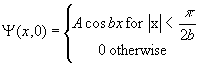a)      Determine the constant A such that the wavefunction Y(x,0) is normalized.

b)      What is the probability for finding the particle in the interval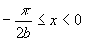?

c)      What is the momentum amplitude for the wavefunction?

d)      For what value of the momentum is the momentum probability maximum?

e)      What is the probability for finding the momentum in an interval dp around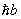?

f)        Evaluate the uncertainty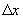.

g)      Evaluate the uncertainty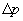.

## 4.                  [2 pt] Classical quantities in quantum mechanics: velocity and momentum

Ohanian, #2.23, p. 56